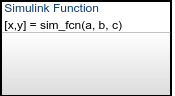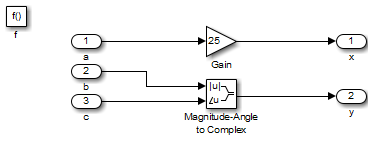Documentation

## Define a Simulink Function in a Stateflow Chart

A Simulink® function is a graphical object that you fill with Simulink blocks and call in the actions of states and transitions. Simulink functions are supported only in Stateflow® charts in Simulink models. For more information, see Reuse Simulink Components in Stateflow Charts.

1. Click the Simulink function icon in the Stateflow Editor toolbar:2. Move your pointer to the location for the new Simulink function in your chart and click to insert the function box.

### Tip

You can also drag the function from the toolbar.

3. Enter the function signature.

The function signature specifies a name for your function and the formal names for the arguments and return values. A signature has this syntax:

``[r_1, r_2,..., r_n] = simfcn(a_1, a_2,..., a_n)``

where `simfcn` is the name of your function, `a_1`, `a_2`, ..., `a_n` are formal names for the arguments, and `r_1`, `r_2`, ..., `r_n` are formal names for the return values.

### Note

This syntax is the same as what you use for graphical functions, truth tables, and MATLAB® functions. You can define arguments and return values as scalars, vectors, or matrices of any data type.

4. Click outside the function box.

The following example shows a Simulink function that has the name `sim_fcn`, which takes three arguments (`a`, `b`, and `c`) and returns two values (`x` and `y`).### Note

You can also create and edit a Simulink function by using API methods.

Follow these steps to define the subsystem elements of the Simulink function:

1. Double-click the Simulink function box.

The contents of the subsystem appear: input and output ports that match the function signature and a single function-call trigger port.

3. Connect the input and output ports to each block.

### Note

You cannot delete the trigger port in the function.

The following example shows the subsystem elements for a Simulink function.### Task 3: Configure the Function Inputs

1. Configure the input ports.

1. Double-click an input port to open the Block Parameters dialog box.

2. In the Signal Attributes pane, enter the size and data type.

For example, you can specify a size of `[2 3]` for a 2-by-3 matrix and a data type of `uint8`.

2. Click .

### Note

An input port of a Simulink function cannot inherit size or data type. Therefore, you define the size and data type of an input that is not scalar data of type `double`. However, an output port can inherit size and data type.

## Related Topics

##### SupportGet trial now